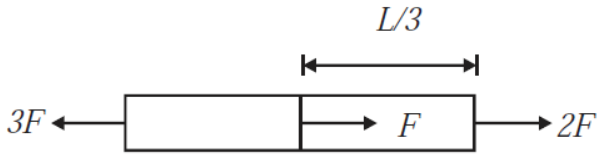Select Chapter Topics:# One end of a uniform wire of length L and of weight W is attached rigidly to a point in the roof and a weight W1 is suspended from its lower end. If A is the area of cross-section of the wire , the stress in the wire at a height 3L/4 from its lower end is: 1.  $\frac{\mathrm{W}+{\mathrm{W}}_{1}}{\mathrm{A}}$ 2.  $\frac{4\mathrm{W}+{\mathrm{W}}_{1}}{3\mathrm{A}}$ 3.  $\frac{3\mathrm{W}+{\mathrm{W}}_{1}}{4\mathrm{A}}$ 4.  $\frac{\frac{3}{4}\mathrm{W}+{\mathrm{W}}_{1}}{\mathrm{A}}$Subtopic:  Stress - Strain |
69%
To view explanation, please take trial in the course.
NEET 2023 - Target Batch - Aryan Raj Singh
Hints

The bulk modulus of water is $2×{10}^{9}$ $\mathrm{N}/{\mathrm{m}}^{2}$. The increase in pressure required to decrease the volume of water sample by $$0.1$$% is:
1. $$4\times 10^{6}$$ N/m2
2. $$2\times 10^{6}$$ N/m2
3. $$2\times 10^{8}$$ N/m2
4. $$8\times 10^{6}$$ N/m2Subtopic:  Shear and bulk modulus |
84%
From NCERT
To view explanation, please take trial in the course.
NEET 2023 - Target Batch - Aryan Raj Singh
Hints
To view explanation, please take trial in the course.
NEET 2023 - Target Batch - Aryan Raj Singh

To break a wire, a force of ${10}^{6}$ $N/{m}^{2}$ is required. If the density of the material is $3×{10}^{3}$ $kg/{m}^{3}$, then the length of the wire which will break by its own weight will be:

1. 34 m

2. 30 m

3. 300 m

4. 3 mSubtopic:  Stress - Strain |
62%
To view explanation, please take trial in the course.
NEET 2023 - Target Batch - Aryan Raj Singh
Hints
To view explanation, please take trial in the course.
NEET 2023 - Target Batch - Aryan Raj Singh

The length of elastic string, obeying Hooke's law is ${l}_{1}$ metres when the tension is 4N, and ${l}_{2}$ metres when the tension is 5N. The length in metres when the tension is 0 N will be-

1. $5{l}_{1}-4{l}_{2}$

2. $5{l}_{2}-4{l}_{1}$

3. $9{l}_{1}-8{l}_{2}$

4. $9{l}_{2}-8{l}_{1}$Subtopic:  Hooke's Law |
63%
To view explanation, please take trial in the course.
NEET 2023 - Target Batch - Aryan Raj Singh
Hints
To view explanation, please take trial in the course.
NEET 2023 - Target Batch - Aryan Raj Singh

Two wires are made of the same material and have the same volume. The first wire has a cross-sectional area A and the second wire has a cross-sectional area 3A. If the length of the first wire is increased by $∆\mathrm{l}$ on applying a force F, how much force is needed to stretch the second wire by the same amount?

 1 9F 2 6F 3 4F 4 FSubtopic:  Young's modulus |
75%
From NCERT
NEET - 2018
To view explanation, please take trial in the course.
NEET 2023 - Target Batch - Aryan Raj Singh
Hints
To view explanation, please take trial in the course.
NEET 2023 - Target Batch - Aryan Raj Singh

Copper of fixed volume 'V' is drawn into a wire of length 'l'. When this wire is subjected to a constant force 'F', the extension produced in the wire is 'Δl'. Which of the following graph is a straight line?

1. $∆\mathrm{l}$ $\mathrm{vs}$ $\frac{1}{\mathrm{l}}$

2. $∆\mathrm{l}$ $\mathrm{vs}$ ${\mathrm{l}}^{2}$

3. $∆\mathrm{l}$ $\mathrm{vs}$ $\frac{1}{{\mathrm{l}}^{2}}$

4. $∆\mathrm{l}$ $\mathrm{vs}$ $\mathrm{l}$Subtopic:  Young's modulus |
70%
From NCERT
AIPMT - 2014
To view explanation, please take trial in the course.
NEET 2023 - Target Batch - Aryan Raj Singh
Hints
To view explanation, please take trial in the course.
NEET 2023 - Target Batch - Aryan Raj Singh

Overall changes in volume and radius of a uniform cylindrical steel wire are 0.2% and 0.002% respectively when subjected to some suitable force. Longitudinal tensile stress acting on the wire is:
($\mathrm{Y}=2.0×{10}^{11}$ ${\mathrm{Nm}}^{-2}$)

1.  $3.2×{10}^{11}$ ${\mathrm{Nm}}^{-2}$

2.  $3.2×{10}^{7}$ ${\mathrm{Nm}}^{-2}$

3.  $3.6×{10}^{9}$ ${\mathrm{Nm}}^{-2}$

4.  3.9$×{10}^{8}$ ${\mathrm{Nm}}^{-2}$Subtopic:  Young's modulus |
From NCERT
To view explanation, please take trial in the course.
NEET 2023 - Target Batch - Aryan Raj Singh
Hints
To view explanation, please take trial in the course.
NEET 2023 - Target Batch - Aryan Raj Singh

A 1000 kg lift is tied with metallic wires of maximum safe stress of 1.4 $×$ 108 N m-2. If the maximum acceleration of the lift is 1.2 m s-2, then the minimum diameter of the wire is:
1. 1 m

2. 0.1 m

3. 0.01 m

4. 0.001 mSubtopic:  Stress - Strain |
56%
From NCERT
To view explanation, please take trial in the course.
NEET 2023 - Target Batch - Aryan Raj Singh
Hints
To view explanation, please take trial in the course.
NEET 2023 - Target Batch - Aryan Raj Singh

A wire can sustain a weight of 10 kg before breaking. If the wire is cut into two equal parts, then each part can sustain a weight of:

 1 2.5 kg 2 5 kg 3 10 kg 4 15 kgSubtopic:  Stress - Strain |
71%
From NCERT
To view explanation, please take trial in the course.
NEET 2023 - Target Batch - Aryan Raj Singh
Hints
To view explanation, please take trial in the course.
NEET 2023 - Target Batch - Aryan Raj Singh

A uniform cylinder rod of length L, cross-sectional area A and Young's modulus Y is acted upon by the forces, as shown in the figure. The elongation of the rod is:1. $\frac{3FL}{5AY}$

2. $\frac{2FL}{5AY}$

3. $\frac{2FL}{8AY}$

4. $\frac{8FL}{3AY}$Subtopic:  Young's modulus |
58%
From NCERT
To view explanation, please take trial in the course.
NEET 2023 - Target Batch - Aryan Raj Singh
Hints